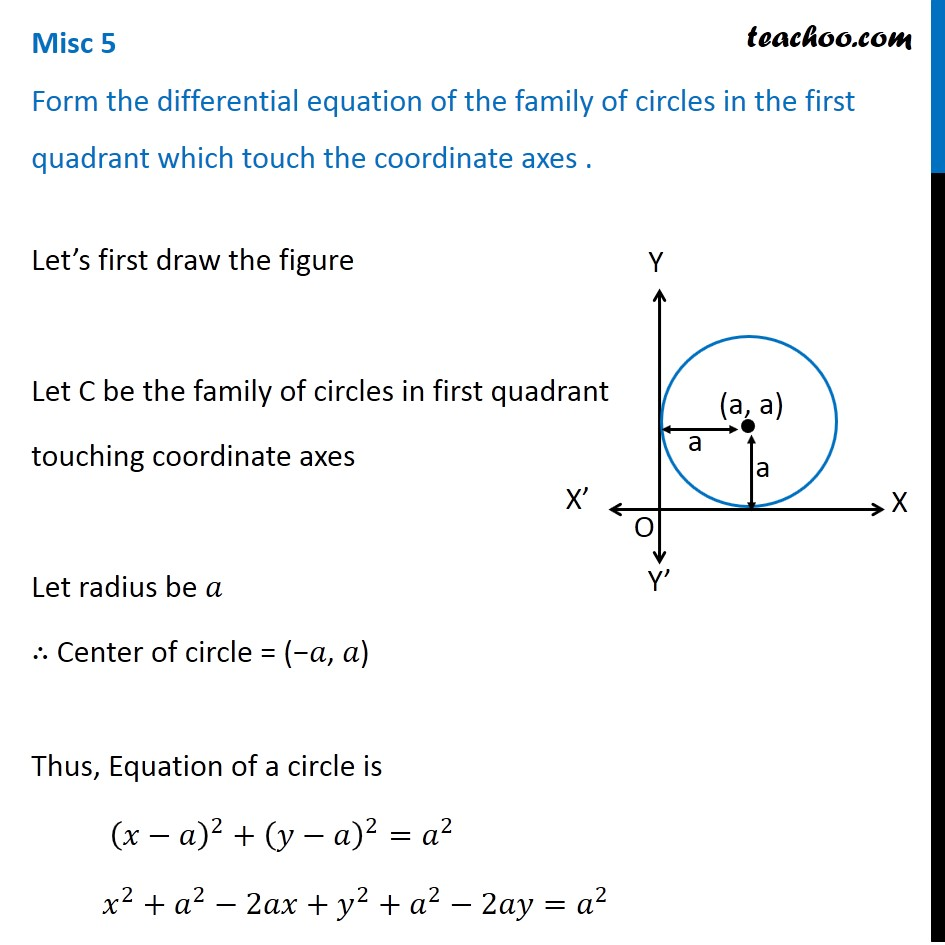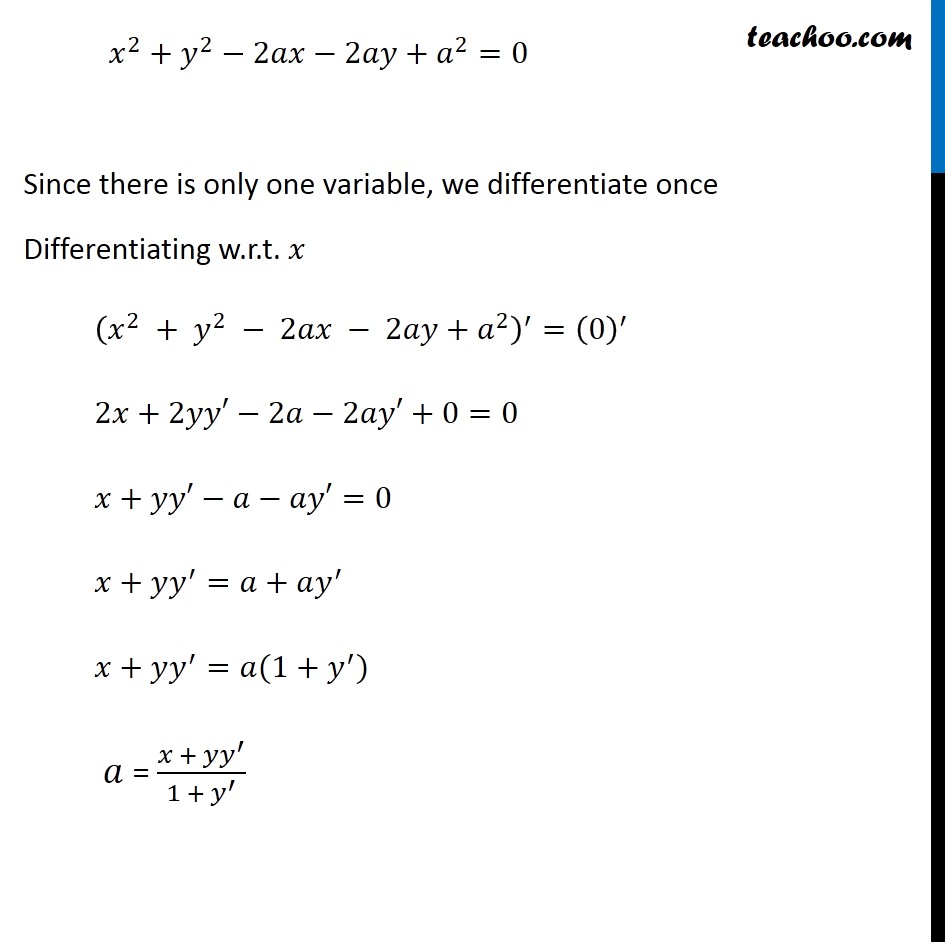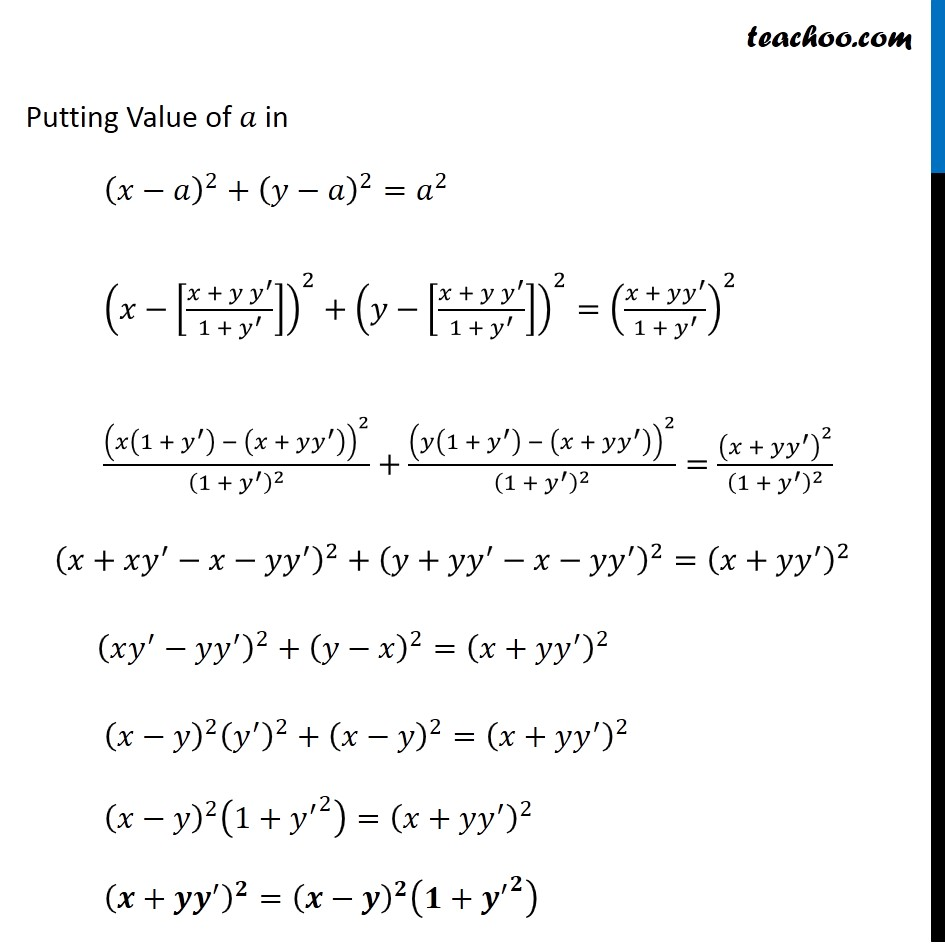Miscellaneous

Chapter 9 Class 12 Differential Equations
Serial order wiseLearn in your speed, with individual attention - Teachoo Maths 1-on-1 Class

### Transcript

Question 2 Form the differential equation of the family of circles in the first quadrant which touch the coordinate axes . Let’s first draw the figure Let C be the family of circles in first quadrant touching coordinate axes Let radius be 𝑎 ∴ Center of circle = (−𝑎, 𝑎) Thus, Equation of a circle is (𝑥−𝑎)^2+(𝑦−𝑎)^2=𝑎^2 𝑥^2+𝑎^2−2𝑎𝑥+𝑦^2+𝑎^2−2𝑎𝑦=𝑎^2 𝑥^2+𝑦^2−2𝑎𝑥−2𝑎𝑦+𝑎^2=0 Since there is only one variable, we differentiate once Differentiating w.r.t. 𝑥 (𝑥^2 + 𝑦^2 − 2𝑎𝑥 − 2𝑎𝑦+𝑎^2 )^′=(0)^′ 2𝑥+2𝑦𝑦′−2𝑎−2𝑎𝑦′+0=0 𝑥+𝑦𝑦′−𝑎−𝑎𝑦′=0 𝑥+𝑦𝑦^′=𝑎+𝑎𝑦^′ 𝑥+𝑦𝑦^′=𝑎(1+𝑦^′) 𝑎 = (𝑥 + 𝑦𝑦^′)/(1 + 𝑦^′ ) Putting Value of 𝑎 in (𝑥−𝑎)^2+(𝑦−𝑎)^2=𝑎^2 (𝑥−[(𝑥 + 𝑦 𝑦^′)/(1 + 𝑦^′ )])^2+(𝑦−[(𝑥 + 𝑦 𝑦^′)/(1 + 𝑦^′ )])^2=((𝑥 + 𝑦𝑦^′)/(1 + 𝑦^′ ))^2 (𝑥(1 + 𝑦^′ ) − (𝑥 + 𝑦𝑦^′ ))^2/(1 + 𝑦^′ )^2 +(𝑦(1 + 𝑦^′ ) − (𝑥 + 𝑦𝑦^′ ))^2/(1 + 𝑦^′ )^2 =(𝑥 + 𝑦𝑦^′ )^2/(1 + 𝑦^′ )^2 (𝑥+𝑥𝑦^′−𝑥−𝑦𝑦^′ )^2+(𝑦+𝑦𝑦^′−𝑥−𝑦𝑦^′ )^2=(𝑥+𝑦𝑦^′ )^2 (𝑥𝑦^′−𝑦𝑦^′ )^2+(𝑦−𝑥)^2=(𝑥+𝑦𝑦^′ )^2 (𝑥−𝑦)^2 (𝑦^′ )^2+(𝑥−𝑦)^2=(𝑥+𝑦𝑦^′ )^2 (𝑥−𝑦)^2 (1+〖𝑦^′〗^2 )=(𝑥+𝑦𝑦^′ )^2 (𝒙+𝒚𝒚^′ )^𝟐=(𝒙−𝒚)^𝟐 (𝟏+〖𝒚^′〗^𝟐 )